Next Article in Journal
Analysis of Occupants’ Visual Perception to Refine Indoor Lighting Environment for Office Tasks
Next Article in Special Issue
Current State of Technology of Fuel Cell Power Systems for Autonomous Underwater Vehicles
Previous Article in Journal
New Approach to Fuelization of Herbaceous Lignocelluloses through Simultaneous Saccharification and Fermentation Followed by Photocatalytic Reforming
Previous Article in Special Issue
Evaluation of the Wave Energy Conversion Efficiency in Various Coastal Environments
Open AccessArticle

# Explicit Expressions for Solar Panel Equivalent Circuit Parameters Based on Analytical Formulation and the Lambert W-Function†

byJavier Cubas *,Santiago PindadoandCarlos De Manuel
Instituto Universitario de Microgravedad "Ignacio Da Riva" (IDR/UPM), Escuela Técnica Superior de Ingeniería Aeronáutica y del Espacio (ETSIAE), Universidad Politécnica de Madrid, Pza. del Cardenal Cisneros 3, Madrid 28040, Spain
*
Author to whom correspondence should be addressed.
An early version of this paper was previously presented at the 1st International e-Conference on Energies, 2014, c013; doi:10.3390/ece-1-c013, available online: http://www.sciforum.net/conference/ece-1/paper/2340.
Energies 2014, 7(7), 4098-4115; https://doi.org/10.3390/en7074098
Received: 21 April 2014 / Revised: 18 June 2014 / Accepted: 23 June 2014 / Published: 26 June 2014

## Abstract

Due to the high dependence of photovoltaic energy efficiency on environmental conditions (temperature, irradiation...), it is quite important to perform some analysis focusing on the characteristics of photovoltaic devices in order to optimize energy production, even for small-scale users. The use of equivalent circuits is the preferred option to analyze solar cells/panels performance. However, the aforementioned small-scale users rarely have the equipment or expertise to perform large testing/calculation campaigns, the only information available for them being the manufacturer datasheet. The solution to this problem is the development of new and simple methods to define equivalent circuits able to reproduce the behavior of the panel for any working condition, from a very small amount of information. In the present work a direct and completely explicit method to extract solar cell parameters from the manufacturer datasheet is presented and tested. This method is based on analytical formulation which includes the use of the Lambert W-function to turn the series resistor equation explicit. The presented method is used to analyze commercial solar panel performance (i.e., the current-voltage–I-V–curve) at different levels of irradiation and temperature. The analysis performed is based only on the information included in the manufacturer’s datasheet.

## 1. Introduction

Today, the increasing use of renewable energy sources is not only a fact, but it also represents a great concern of modern society, as the effects of global warming are more and more evident and have spread to almost every corner of planet Earth. Some of these renewable energy sources such as wind energy  or both solar photovoltaic [2,3] and solar thermal , had an enormous development in the recent decades.
Focusing on solar photovoltaic energy current users, there is not a clear and defined user profile, those users ranging from the industrial sector, represented by big power plants, to the private sector represented by citizens who invest in solar panels systems to save costs in terms of electricity consumption. Also, the space sector demands photovoltaic technology, and although it is not as massive as the aforementioned ones, it should be said that it leads the technological advances related to solar cells.
Despite the wide diversity between users, there is a common need among them: to have a better understanding of their photovoltaic systems in order to get the best efficiency from them in terms of costs and revenue. On the other hand, photovoltaic energy is highly dependent on the environmental conditions (temperature, irradiation...) and that complicates its optimization, making the modeling of the panel a necessary tool. Modeling of solar panels is traditionally achieved through numerical fitting to extensive experimental results. However, this is not affordable for small users and it is not practical when a decision among different commercial solar panels/systems has to be made and only the information included in the manufacturers’ datasheets is available. Analytical methods represent a solution to this problem, as they are simpler and only require a small amount of data (frequently included in the datasheet) to model the solar panel behavior.
In the present work an analytical methodology to model the behavior (output current, I, and output voltage, V) of a photovoltaic device (cell or solar panel) is presented. It is based on the use of an equivalent circuit, which is an extended and accurate way to model solar panels. The present work is part of a larger research related to the UPMSat-2 (Universidad Politécnica de Madrid Satélite 2) satellite mission. In previous studies some analytical methods for parameter calculation were successfully developed, the aim now being to simplify the equations (with more sophisticated mathematical tools such as the Lambert function [5,6]), and show how these algorithms can be used in a practical application: to modeling a commercial solar panel at different levels of irradiation and temperature. This is a necessity for a correct power optimization, or to include polar panel modeling in bigger electric power simulations (e.g., maximum power point tracking (MPPT) models), but with current methods it is not possible to do it with a small amount of information, and in a way accurate and simple at the same time. This method aims to solve this problem and enhance analysis capabilities to any user of solar energy.

## 2. Methods

As it is well known, ideal solar cells behave like a current source connected in parallel with a diode [7,8,9]. This ideal model is completed with resistors to represent the losses and sometimes with additional diodes that takes into account other phenomena [10,11]. The most popular circuit equivalent to a solar cell/panel is shown in Figure 1, it includes a current source, one diode and two resistors: one in series and one in parallel [12,13,14,15,16,17,18,19]. Each element included in the equivalent circuit implies one parameter that has to be determined (two in the case of the diode whose behavior is represented by the Shockley equation ). Therefore, five parameters need to be calculated when using this method [21,22,23,24,25,26,27,28,29,30,31,32,33]. The current-voltage curve of a solar cell or panel, hereinafter the I-V curve (see Figure 2), is quite well reproduced by this simple equivalent circuit. Three points of the I-V curve are also indicated in Figure 2: short circuit, maximum power, and open circuit points. These representative points are, together with their variation as a function of the temperature, the normal information included in manufacturers’ datasheets.
Figure 1. Equivalent circuit of a solar panel.
Figure 1. Equivalent circuit of a solar panel.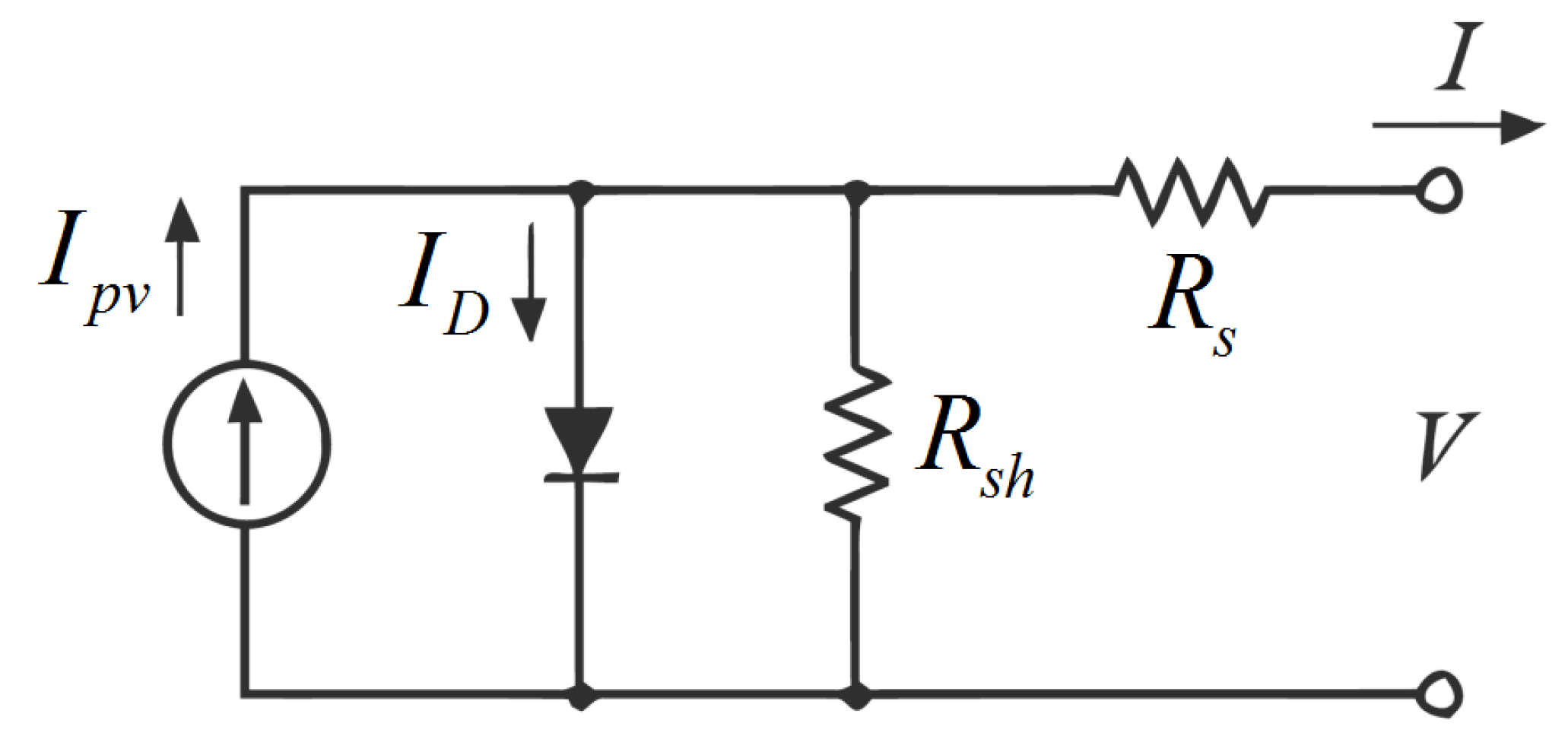Figure 2. I-V curve of a solar panel. The three characteristic points (short circuit, maximum power, and open circuit points) are indicated on the curve.
Figure 2. I-V curve of a solar panel. The three characteristic points (short circuit, maximum power, and open circuit points) are indicated on the curve.The I-V behavior of the circuit model formed by one diode and two resistors (Figure 1) is defined by the following equation :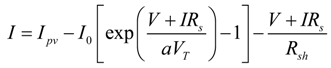where Ipv is the photocurrent delivered by the constant current source; I0 is the reverse saturation current corresponding to the diode; Rs is the series resistor that takes into account losses in cell solder bonds, interconnection, junction box, etc.; Rsh is the shunt resistor that takes into account the current leakage through the high conductivity shunts across the p-n junction; and a is the ideality factor that takes into account the deviation of the diodes from the Shockley diffusion theory. VT is not an unknown parameter; it is the thermal voltage of the diode and depends on the charge of the electron, q, the Boltzmann constant, k; the number of cells in series, n; and the temperature, T:As said, the parameters of the equivalent circuit have to be adjusted to fit the behavior of the equivalent circuit to the solar cell/panel testing results. This adjustment can be done numerically [15,16,34,35,36,37,38,39] or analytically [9,39,40,41,42]; based on the data resulting from I-V curves experimentally measured, or based only on the data available from the manufacturers’ datasheets.

#### 2.1. Parameter Calculation

In this paper only manufacturer’s data is considered to be available for parameter calculation, this is the common situation that faces a regular user from the private sector. In general, commercial datasheets only include information about the three characteristic points of the I-V curve for different temperatures, from this three points it is possible to extract four boundary conditions . As these conditions are not enough to determine the five parameters of the equivalent circuit, in absence of more information, some authors propose to estimate the parameter a, in order to reduce the number of parameters to four . This parameter is the preferred to be estimated because its value is bound to a small range, it usually is in the bracket [1, 1.5] for a single junction solar cell [19,44,45]. Applying the four boundary conditions to the equivalent circuit Equation (1) it is possible to obtain the following equations [21,46]:
• Short circuit equation: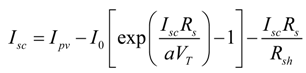• Open circuit equation:• Maximum power point circuit equation:• Zero derivative for the power at maximum power point circuit equation: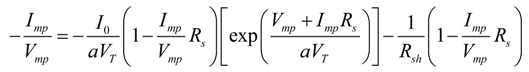With some minor and justified simplifications (that is, taking into account order of magnitude of the different terms in the equations), the above equations can be simplified to the following :
• Equation for Rs:• Equation for Rsh: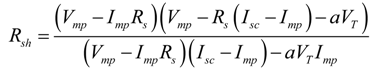• Equation for I0:• Equation for Ipv:By estimating parameter a, and solving the previous system, it is possible to determine the values of the equivalent circuit parameters simply, straight forward, and quite accurately. However, although the mathematical system is decoupled, Equation (7) is still implicit for Rs. This equation is not difficult to solve with numerical calculation programs (e.g., Matlab or Maple). Nevertheless, it is possible to transform it into an explicit equation using the Lambert W-function . The Lambert W-function, W(z), is defined as:
z = W (z) eW(z)
where z is any complex number. This function is not injective; in the real variable x the relation W is defined only for x ≥ −1/e and is double valued in the bracket [−1/e, 0], the two branches of the function being expressed as W0(x) for W(x) ≥ −1 and W−1(x) for W(x) ≤ −1 in the aforementioned bracket. The general strategy to apply the Lambert W-function in solving exponential equations is to use the following equivalence:
X = YeYY = W(X)
Going back to the equivalent circuit equations, Equation (7) can be rewritten as follows:Using the mathematical equivalence from Equation (12), Equation (13) turns into: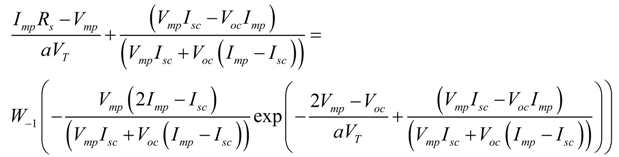where W−1 is the negative branch of the Lambert W-function (as the left part of Equation (14) is lower than −1 for typical cells and solar panels). And then, an explicit expression for Rs is obtained:
Rs = A(W−1(Bexp(C)) − (D + C))
where:In recent years, the Lambert W-function has become a common mathematical tool in solar cell/panel equivalent circuit extraction parameters, as it is quite useful for solving exponential equations like the expression of the I-V curve. Commonly, the mathematical relation (12) is directly applied to Equation (1) in order to obtain explicit equations for the solar cell/panel output current or voltage. This method is helpful for the determination of equivalent circuit parameters through numerical methods, that is, when testing data is available and a curve fitting is performed [23,48]. However, it is not convenient to use the Lambert W-function to transform Equation (1) if the aim is to solve the problem analytically, because it is not possible to obtain a decoupled system of equations . In this particular case, the approach used is different, it consists in using the Lambert W-function as the last step of the procedure; clearing Rs from the remaining implicit equation after obtaining explicit equations for the other parameters. Then, a fully decoupled set of explicit equations for the equivalent circuit parameters can be obtained. Data from two solar cells, included in Table 1, have been used to validate the results obtained following the procedure described in the text. Also, the accuracy of the method can be checked. In Table 2, the values of the equivalent circuit parameters obtained are compared to the ones from , obtained through a curve fitting technique and used as reference for the parameter a, and to the results from another method based on the Lambert W-function, with the difference that this other one is iterative (and not explicit) .
Table 1. Characteristic of blue solar cells and grey solar cells .
Table 1. Characteristic of blue solar cells and grey solar cells .
Blue Solar CellGrey Solar Cell
a1.51 ± 0.07a1.72 ± 0.08
Imp (A)0.0934Imp (A)0.485
Vmp (V)0.433Vmp (V)0.387
Isc (A)0.1023Isc (A)0.561
Voc (V)0.536Voc (V)0.524
Tr (K)300Tr (K)307
Table 2. Characteristic of blue solar cells and grey solar cells .
Table 2. Characteristic of blue solar cells and grey solar cells .
Blue Solar CellGrey Solar Cell
Equivalent Circuit Parameters Benchmark Iterative Method Present MethodEquivalent Circuit ParametersBenchmark Iterative Method Present Method
Rs (Ω)0.07 ± 0.0090.06710.0652Rs (Ω)0.08 ± 0.010.07840.0781
Rsh (Ω)1000 ± 509771093Rsh (Ω)26 ± 126.0926.25
Ipv (A)0.1023 ± 0.00050.10230.1023Ipv (A)0.5625 ± 0.00050.5610.5627
I0 (A)110 ± 50 × 10−9111 × 10−9111 × 10−9I0 (A)6 ± 3 × 10−65.6 × 10−65.4 × 10−6

#### 2.2. Parameter Dependence on Environmental Conditions

Environmental conditions, such as temperature or irradiation variations, modify the behavior of photovoltaic devices, that is, the I-V curve. Therefore, those variations affect the boundary conditions of the equations and, accordingly, the value of the equivalent circuit parameters.
When temperature raises, the current increases and the voltage decreases, and as a result, the maximum power point of the I-V curve also decreases. These variations are approximately linear with temperature, and most solar panel manufacturers include the rate of variation of the characteristic I-V curve points in the datasheets, either as absolute increase or as percent variation. In the second case the variations with temperature T of current and voltage levels at open circuit, maximum power, and short circuit points can be expressed as: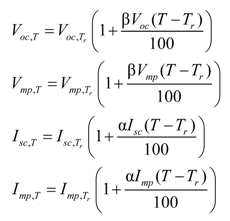where Tr is the reference temperature; βVoc and βVmp are respectively the percentage variation of the open circuit and maximum power point voltages when the temperature increases one degree; finally, αIsc and αImp are the percentage variation of the short circuit and maximum power point currents when the temperature increases one degree. Some manufacturers include the percentage variation of the maximum power with temperature, γ, instead of specific variations for current and voltage at that point. If αImp or βVmp (one of them) is missing, then the variation of the boundary condition can be calculated using the parameter γ and the remaining condition, for example: Imp value in terms of γ and βVmp is: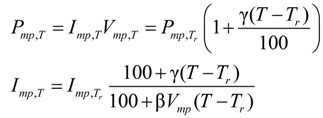On the other hand, if both αImp and βVmp are not included in the manufacturer’s datasheet and only the parameter γ is provided, it can be assumed that βVmp ≈ βVoc before using Equation (18). With these relations it is possible to directly relate the equivalent circuit parameters and the rate of variations with T [22,44]. Nevertheless, a different approximation is used in the present work: the equivalent circuit parameters are calculated for different temperatures with Equation (17), using the values of the characteristic points at those temperatures. Then, the variation of the parameters as a function of temperature T is empirically determined based on these results. This procedure is very accurate, as the calculated equivalent circuits directly meet the manufacturers’ data.
Any information regarding the dependence of the solar cell/panel on irradiation levels is commonly not included in manufacturers’ datasheets, as this dependence is well known and easy to be determined. The shape of the I-V curve is essentially invariant with intensity in ranges around one solar constant, temperature affects Isc linearly and Voc exponentially, whereas Rs remains not affected for temperature variations . Those conditions lead to the following equation :where G is the irradiance on the cell/solar panel, Ipv,G is the photocurrent delivered by the current source of the equivalent circuit, and Gr and Ipv,Gr are the reference values. When data about irradiation dependence is available, it is generally shown as experimental I-V curves, in this case it would be possible to extract information about the characteristic points graphically, and therefore define the dependence on irradiation with a similar procedure to the one followed in case of temperature variations.

## 3. Results and Discussion

In this Section, the equivalent circuits of two commercial solar panels (MSP290AS-36.EU and MSMD290AS-36 by München Solarenergie GmbH, Neu-Ulm, Germany), are calculated as an example of application of the above method. For that calculation only data provided by the manufacturer is used [52,53], see Table 3. The objective is to obtain an equivalent circuit that reproduces the solar panel behavior for any irradiation and temperature levels. The first step is to estimate the ideality factor, a. Some authors propose a generic value in the bracket [1, 1.5] for every kind of cell , although different technologies are associated with different values of the aforementioned ideality factor at standard conditions. In  a = 1.2 is suggested for monocrystalline silicon cells, whereas a = 1.3 is suggested for polycrystalline ones. This parameter is normally considered independent of temperature. However, some studies indicate a decreasing linearity with temperature . In any case, the lack of accuracy produced when considering the ideality factor as constant is generally reduced, given that variations of this parameter only affects the curvature of the I-V curve. Nevertheless, it should also be pointed out that a not physically possible solution for the equivalent circuit parameters (negative values of the resistors), can be obtained if a value of the ideality factor too detached from the real one is chosen, as the aforementioned curvature is incompatible with the boundary conditions (i.e., the characteristic points). The possibility of those not physically possible solutions increases in large temperature ranges, if the same value of the ideality factor is taken for the lower and the higher temperatures. In those cases a decreasing linear variation law with temperature for parameter a could be included , or alternatively just values of parameter a that result in a valid solution for most of the temperature interval under consideration could be selected. In the present study an ideality factor of a = 1.1, has been chosen for the silicon cell panels. Given that the ideality factor a decreases as temperature rises, this slightly lower value of a with respect to the figures assumed by other authors , increases the highest temperature for which physically possible solutions exist. The procedure to extract the equivalent circuit parameters is therefore as follows:
• Estimate the value of the parameter a. In this method this parameter is considered as a constant, independent of the temperature and the irradiation. In this case the chosen value is a = 1.1.
• Calculate Vt for the panel at that temperature level with Equation (2).
• Determine the boundary conditions at that temperature and irradiation level using manufacturer’s data and Equations (17) and/or (18).
• Calculate Rs with Equation (15).
• Calculate Rsh with Equation (8).
• Calculate I0 with Equation (9).
• Calculate Ipv with Equation (10).
Table 3. Characteristic points of MSP290AS-36.EU and MSMD290AS-36 solar panels (München Solarenergie GmbH), included in the manufacturer datasheets [52,53] at STC (Standard Test Conditions) (1000 W/m2 irradiance, 25 °C cell temperature, AM1.5g spectrum according to EN 60904-3).
Table 3. Characteristic points of MSP290AS-36.EU and MSMD290AS-36 solar panels (München Solarenergie GmbH), included in the manufacturer datasheets [52,53] at STC (Standard Test Conditions) (1000 W/m2 irradiance, 25 °C cell temperature, AM1.5g spectrum according to EN 60904-3).
MSP290AS-36.EU (Multicrystalline)MSMD290AS-36.EU (Monocrystalline)
n72Tr (°C)25n72Tr (°C)25
Pmp (W)290γ (%/°C)−0.45Pmp (W)290γ (%/°C)−0.44
Imp (A)7.82αImp (%/°C)-Imp (A)7.70αImp (%/°C)-
Vmp (V)37.08βVmp (%/°C)−0.35Vmp (V)37.66βVmp (%/°C)−0.35
Isc (A)8.37αIsc (%/°C)+0.04Isc (A)8.24αIsc (%/°C)+0.04
Voc (V)44.32βVoc (mV/°C)−0.33Voc (V)44.68βVoc (mV/°C)−0.31
The values of circuit parameters obtained at AM1.5g (1000 W/m2) and Tr = 25 °C, are included in Table 4.
Table 4. Parameters of MSP290AS-36.EU and MSMD290AS-36.EU (München Solarenergie GmbH) solar panels equivalent circuits at STC (1000 W/m2 irradiance, 25 °C cell temperature, AM1.5g spectrum).
Table 4. Parameters of MSP290AS-36.EU and MSMD290AS-36.EU (München Solarenergie GmbH) solar panels equivalent circuits at STC (1000 W/m2 irradiance, 25 °C cell temperature, AM1.5g spectrum).
MSP290AS-36.EU (Multicrystalline)MSMD290AS-36.EU (Monocrystalline)
a1.10a1.10
Ipv (A)8.37Ipv (A)8.24
I0 (A)2.86 × 10−9I0 (A)2.36 × 10−9
Rs (Ω)0.162Rs (Ω)0.130
Rsh (Ω)331Rsh (Ω)316
In order to spread the use of the equivalent circuit to any temperatures inside the range 15 to 85 °C (this bracket includes most of the operational temperatures; it should be taken also into account that the temperature of the panel is higher than the ambient temperature during operation), the process is repeated for temperatures in that range. Parameter values, showing their variation with temperature, are shown in Figure 3. In the figure it can be observed how parameter Rs turns negative for high temperatures (the value of parameter Rsh could also turn negative under some conditions). As previously suggested, those mathematically correct but not physically valid solutions at high temperatures are derived in cases where the boundary conditions (slopes of the I-V curve at short circuit and open circuit points), are not compatible with the curvature determined by the chosen ideality factor.
Numerical expressions for the circuit parameters as a function of the temperature, T, are then derived from this results using polynomial approximation with the least squares method:
• For MSP290AS-36.EU:
Ipv (T) = 8.37 + 3.62·10−3T − 3.38·10−6T2 − 7.58·10−8T3
Rs (T) = 1.62·10−1 − 3.21·10−3T + 7.05·10−7T2 − 3.01·10−8T3
Rsh (T) = 1/(3.03·10−3 + 2.65·10−4T + 1.50·10−6T2 + 1.56·10−8T3)
I0 (T) = exp(−1.97·101 + 1.44·10−1T − 4.80·10−4T2 + 1.15·10−6T3)
• For MSMD290AS-36.EU:
Ipv (T) = 8.24 + 3.49·10−3T − 1.68·10−6T2 − 2.41·10−8T3
Rs (T) = 1.30·10−1 − 1.97·10−3T + 2.53·10−6T2 − 1.07·10−8T3
Rsh (T) = 1/(3.18·10−3 + 2.33·10−4T + 1.27·10−6T2 + 1.33·10−8T3)
I0 (T) = exp(−1.98·101 + 1.41·10−1T − 4.69·10−4T2 + 1.13·10−6T3)
The irradiation variation only affects the parameter Ipv and can be described with Equation (19), including this variation the following equations can be then derived for the five parameters of the equivalent circuit:
• For MSP290AS-36.EU:• For MSMD290AS-36.EU: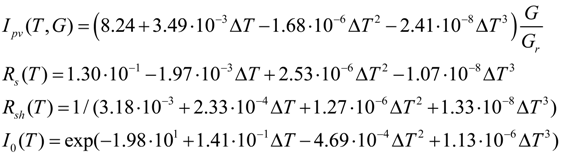Figure 3. Calculated values (using the explained methodology) of the equivalent circuit parameters Rs, Rsh, Ipv, and I0, for multicrystalline (MSP290AS-36.EU) and monocrystalline (MSMD290AS-36.EU) solar cell panels as a function of the temperature, T. Calculated points are indicated with symbols whereas the polynomial approximations fitted to those data (Equations (20) and (21)) have been included in each case as solid lines.
Figure 3. Calculated values (using the explained methodology) of the equivalent circuit parameters Rs, Rsh, Ipv, and I0, for multicrystalline (MSP290AS-36.EU) and monocrystalline (MSMD290AS-36.EU) solar cell panels as a function of the temperature, T. Calculated points are indicated with symbols whereas the polynomial approximations fitted to those data (Equations (20) and (21)) have been included in each case as solid lines.## 4. Experimental Section

In this Section the behavior of the calculated equivalents circuits is analyzed. The aim is to check if the calculated circuit models reproduce the experimental data of the solar panels included in the manufacturer’s datasheet. In order to produce results, the equivalent circuits of the solar panels have been modeled using LTSpice (Linear Technology Corporation, Milpitas, CA, USA), a Simulation Program with Integrated Circuit Emphasis (SPICE) software: Obviously, the mentioned circuits are characterized by the parameters already calculated in Section 3, see Figure 4 and Figure 5. Several simulations have been performed at different temperature and irradiation levels, calculating the I-V curve in each case. The results thereof are included in Figure 6, Figure 7, Figure 8 and Figure 9.
Figure 4. Equivalent circuit of MSP290AS-36.EU (multicrystalline).
Figure 4. Equivalent circuit of MSP290AS-36.EU (multicrystalline).Figure 5. Equivalent circuit of MSMD290AS-36.EU (monocrystalline).
Figure 5. Equivalent circuit of MSMD290AS-36.EU (monocrystalline).Figure 6. I-V curves of panel MSP290AS-36.EU simulated with LTSpice calculation of the equivalent circuits for different irradiation levels, from 200 W/m2 to 1000 W/m2, at 25 °C.
Figure 6. I-V curves of panel MSP290AS-36.EU simulated with LTSpice calculation of the equivalent circuits for different irradiation levels, from 200 W/m2 to 1000 W/m2, at 25 °C.Figure 7. Power curves of panel MSP290AS-36.EU simulated with LTSpice calculation of the equivalent circuits for different temperature levels, from 15 °C to 70 °C, with 1000 W/m2 irradiation level.
Figure 7. Power curves of panel MSP290AS-36.EU simulated with LTSpice calculation of the equivalent circuits for different temperature levels, from 15 °C to 70 °C, with 1000 W/m2 irradiation level.Figure 8. I-V curves of panel MSMD290AS-36.EU simulated with LTSpice calculation of the equivalent circuits for different irradiation levels, from 200 W/m2 to 1000 W/m2, at 25 °C.
Figure 8. I-V curves of panel MSMD290AS-36.EU simulated with LTSpice calculation of the equivalent circuits for different irradiation levels, from 200 W/m2 to 1000 W/m2, at 25 °C.Finally, the results of the simulations performed reproduce with high accuracy the data from the solar panel datasheets regarding the characteristic points’ dependence on the temperature as can be seen in Figure 10, where the manufacturer experimental information about the boundary conditions, Equations (17) and (18), are compared with the characteristic points of the equivalent circuit calculated.
Figure 9. Power curves of panel MSMD290AS-36.EU simulated with LTSpice calculation of the equivalent circuits for different temperature levels, from 15 °C to 85 °C, with 1000 W/m2 irradiation level.
Figure 9. Power curves of panel MSMD290AS-36.EU simulated with LTSpice calculation of the equivalent circuits for different temperature levels, from 15 °C to 85 °C, with 1000 W/m2 irradiation level.Figure 10. Variation of multicrystalline (MSP290AS-36.EU) and monocrystalline (MSMD290AS-36.EU) solar panel characteristic data, Isc, Voc, Pmp, and Vmp as a function of the temperature, T. Symbols represent the characteristic points of calculated equivalent circuits (parameters defined in Equations (22) and (23)), whereas solid lines represent the experimental temperature dependence of these points included in the manufacturer datasheet (see Table 3 and Equations (17) and (18)).
Figure 10. Variation of multicrystalline (MSP290AS-36.EU) and monocrystalline (MSMD290AS-36.EU) solar panel characteristic data, Isc, Voc, Pmp, and Vmp as a function of the temperature, T. Symbols represent the characteristic points of calculated equivalent circuits (parameters defined in Equations (22) and (23)), whereas solid lines represent the experimental temperature dependence of these points included in the manufacturer datasheet (see Table 3 and Equations (17) and (18)).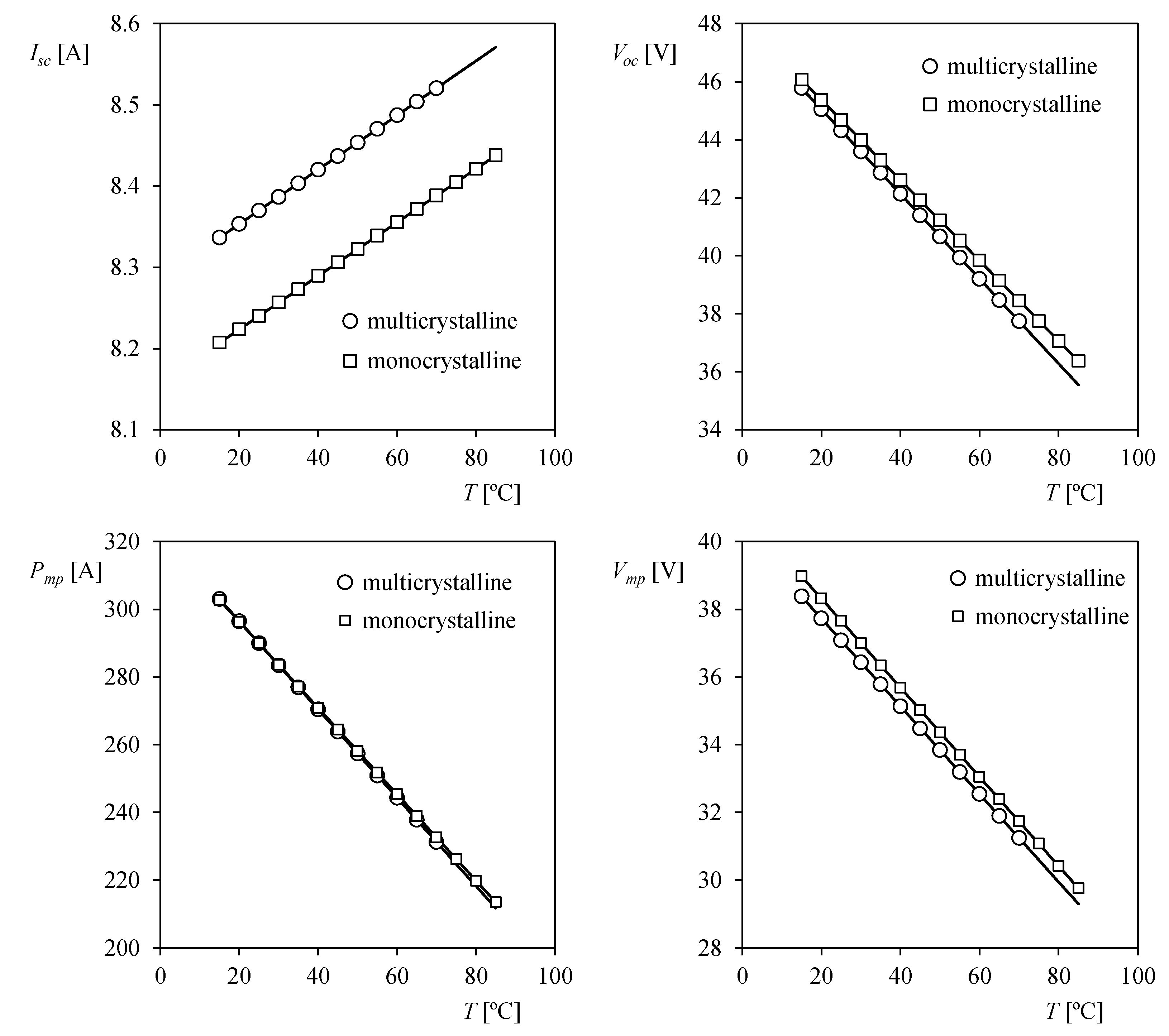## 5. Conclusions

In the present work a simple but accurate method to simulate the performances of a photovoltaic device for different working conditions is presented. The method is based in the analytical determination of the parameters of an equivalent circuit. Using the presented methodology it is possible to construct a realistic model of a solar panel that reproduces the experimental data provided by the manufacturer in the datasheet, including variations at different temperatures and irradiations. The method is explicit, non-iterative and straight forward; no iterations or initial values for the parameters are needed. Only data typically included in manufacturers’ datasheets are required. There are several possible applications for this method. From the authors’ point of view, its simplicity and accuracy would make it interesting for end users with little calculation and testing resources. Also, the procedure described in the present work seems to be very appropriate for analysis that imply profuse calculations of equivalent circuits or for determination of initial values for numerical methods. Finally, an interesting application of this method is to construct realistic models of solar panels that can be used in simulations of MPPT algorithms.

## Acknowledgments

The present work is part the research framework associated to the design and construction of the UPMSat-2 satellite at the IDR/UPM Institute (Instituto de Universitario Microgravedad “Ignacio Da Riva”) of the Polytechnic University of Madrid (Universidad Politécnica de Madrid). Authors are indebted to the support from all the staff of the IDR/UPM Institute. Authors are truly grateful to Anna María Ballester and Brian Elder for their kind help in improving the style of the text.

## Author Contributions

Javier Cubas and Santiago Pindado developed the idea behind the present research, carrying also out the literature review and manuscript preparation at early stages. The methodology and analytic procedures were carried out by Javier Cubas. Carlos de Manuel programmed the LTspice models. Final review, including final manuscript corrections, was done by Santiago Pindado. All authors were in charge of data generation/analysis and post-processing of the results.

## Conflicts of Interest

The authors declare no conflict of interest.

## References

1. Aminzadeh, F.; Pindado, S. How has Spain become a leader in thewind energy industry during the last decade? (An analysis of influential factors on the development of wind energy in Spain). In Proceedings of the EWEA Annual Event, Brussels, Belgium, 14–17 March 2011.
2. Vázquez, C.T. Energía Solar Fotovoltaica; Ediciones Ceysa: Madrid, Spain, 2008. (In Spanish) [Google Scholar]
3. Razykov, T.M.; Ferekides, C.S.; Morel, D.; Stefanakos, E.; Ullal, H.S.; Upadhyaya, H.M. Solar photovoltaic electricity: Current status and future prospects. Sol. Energy 2011, 85, 1580–1608. [Google Scholar] [CrossRef]
4. Hernandez, V.R. Solar Thermal Power. History of a Research Success; Protermosolar: Sevilla, Spain, 2010. [Google Scholar]
5. Valluri, S.R.; Jeffrey, D.J.; Corless, R.M. Some applications of the Lambert W function to physics. Can. J. Phys. 2000, 78, 823–831. [Google Scholar]
6. Caillol, J. Some applications of the Lambert W function to classical statistical mechanics. J. Phys. A Math. Gen. 2003, 36. [Google Scholar] [CrossRef]
7. Sze, S.M. Physics of Semiconductor Devices; Wiley-Interscience: New York, NY, USA, 1969. [Google Scholar]
8. Pfann, W.G.; van Roosbroeck, W. Radioactive and Photoelectric p-n Junction Power Sources. J. Appl. Phys. 1954, 25. [Google Scholar] [CrossRef]
9. Prince, M.B. Silicon solar energy converters. J. Appl. Phys. 1955, 26, 534–540. [Google Scholar]
10. Wolf, M.; Rauschenbach, H. Series resistance effects on solar cell measurements. Adv. Energy Convers. 1963, 3, 455–479. [Google Scholar] [CrossRef]
11. Van Dyk, E.E.; Meyer, E.L. Analysis of the effect of parasitic resistances on the performance of photovoltaic modules. Renew. Energy 2004, 29, 333–344. [Google Scholar] [CrossRef]
12. De Blas, M.; Torres, J.; Prieto, E.; Garcı́a, A. Selecting a suitable model for characterizing photovoltaic devices. Renew. Energy 2002, 25, 371–380. [Google Scholar] [CrossRef]
13. Carrero, C.; Rodríguez, J.; Ramírez, D.; Platero, C. Simple estimation of PV modules loss resistances for low error modelling. Renew. Energy 2010, 35, 1103–1108. [Google Scholar] [CrossRef]
14. Zhu, X.-G.; Fu, Z.-H.; Long, X.-M. Sensitivity analysis and more accurate solution of photovoltaic solar cell parameters. Sol. Energy 2011, 85, 393–403. [Google Scholar] [CrossRef]
15. Bätzner, D.L.; Romeo, A.; Zogg, H.; Tiwari, A.N. CdTe/CdS Solar Cell Performance under Low Irradiance. In Proceedings of the 17th European Photovoltaic Solar Energy Conference and Exhibition, Munich, Germany, 22–26 October 2001; pp. 1–4.
16. Kennerud, K.L. Analysis of Performance Degradation in CdS Solar Cells. IEEE Trans. Aerosp. Electron. Syst. 1969, AES-5, 912–917. [Google Scholar] [CrossRef]
17. Charles, J.; Abdelkrim, M.; Muoy, Y.; Mialhe, P. A practical method of analysis of the current-voltage characteristics of solar cells. Sol. Cells 1981, 4, 169–178. [Google Scholar] [CrossRef]
18. De Soto, W.; Klein, S.A.; Beckman, W.A. Improvement and validation of a model for photovoltaic array performance. Sol. Energy 2006, 80, 78–88. [Google Scholar]
19. Carrero, C.; Amador, J.; Arnaltes, S. A single procedure for helping PV designers to select silicon PV modules and evaluate the loss resistances. Renew. Energy 2007, 32, 2579–2589. [Google Scholar] [CrossRef]
20. Shockley, W. The Theory of p-n Junctions in Semiconductors and p-n Junction Transistors. Bell Syst. Tech. J. 1949, 28, 435–489. [Google Scholar] [CrossRef]
21. Cubas, J.; Pindado, S.; Victoria, M. On the analytical approach for modeling photovoltaic systems behavior. J. Power Sources 2014, 247, 467–474. [Google Scholar] [CrossRef]
22. Lineykin, S. Five-Parameter Model of Photovoltaic Cell Based on STC Data and Dimensionless. In Proceedings of the 2012 IEEE 27th Convention of Electronical and Electronics Engineers in Israel, Eilat, Israel, 14–17 November 2012; pp. 1–5.
23. Peng, L.; Sun, Y.; Meng, Z.; Wang, Y.; Xu, Y. A new method for determining the characteristics of solar cells. J. Power Sources 2013, 227, 131–136. [Google Scholar]
24. Peng, L.; Sun, Y.; Meng, Z. An improved model and parameters extraction for photovoltaic cells using only three state points at standard test condition. J. Power Sources 2014, 248, 621–631. [Google Scholar] [CrossRef]
25. Orioli, A.; Di Gangi, A. A procedure to calculate the five-parameter model of crystalline silicon photovoltaic modules on the basis of the tabular performance data. Appl. Energy 2013, 102, 1160–1177. [Google Scholar] [CrossRef][Green Version]
26. Ma, J.; Man, K.L.; Ting, T.O.; Zhang, N.; Guan, S.-U.; Wong, P.W.H. Approximate single-diode photovoltaic model for efficient I-V characteristics estimation. Sci. World J. 2013, 2013, 230471:1–230471:7. [Google Scholar]
27. Ma, J.; Ting, T.O.; Man, K.L.; Zhang, N.; Guan, S.-U.; Wong, P.W.H. Parameter Estimation of Photovoltaic Models via Cuckoo Search. J. Appl. Math. 2013, 2013, 362619:1–362619:8. [Google Scholar]
28. Li, Y.; Huang, W.; Huang, H.; Hewitt, C.; Chen, Y.; Fang, G.; Carroll, D.L. Evaluation of methods to extract parameters from current–voltage characteristics of solar cells. Sol. Energy 2013, 90, 51–57. [Google Scholar] [CrossRef]
29. Dongue, S.B.; Njomo, D.; Tamba, J.G.; Ebengai, L. Modeling of Electrical Response of Illuminated Crystalline Photovoltaic Modules Using Four- and Five-Parameter Models. Int. J. Emerg. Technol. Adv. Eng. 2012, 2, 612–619. [Google Scholar]
30. Ishibashi, K.; Kimura, Y.; Niwano, M. An extensively valid and stable method for derivation of all parameters of a solar cell from a single current-voltage characteristic. J. Appl. Phys. 2008, 103. [Google Scholar] [CrossRef]
31. Lineykin, S.; Averbukh, M.; Kuperman, A. An improved approach to extract the single-diode equivalent circuit parameters of a photovoltaic cell/panel. Renew. Sustain. Energy Rev. 2014, 30, 282–289. [Google Scholar] [CrossRef]
32. Cotfas, D.T.; Cotfas, P.A.; Kaplanis, S. Methods to determine the DC parameters of solar cells: A critical review. Renew. Sustain. Energy Rev. 2013, 28, 588–596. [Google Scholar] [CrossRef]
33. Cotfas, D.T.; Cotfas, P.A.; Ursutiu, D.; Samoila, C. The methods to determine the series resistance and the ideality factor of diode for solar cells-review. In Proceedings of the 2012 13th International Conference on Optimization of Electrical and Electronic Equipment (OPTIM), Brasso, Romania, 24–26 May 2012; pp. 966–972.
34. Easwarakhanthan, T.; Bottin, J.; Bouhouch, I.; Boutrit, C. Nonlinear Minimization Algorithm for Determining the Solar Cell Parameters with Microcomputers. Int. J. Sol. Energy 1986, 4, 1–12. [Google Scholar] [CrossRef]
35. Gow, J.A.; Manning, C.D. Development of a photovoltaic array model for use in power-electronics simulation studies. IEEE Proc. Electr. Power Appl. 1999, 146, 193–200. [Google Scholar] [CrossRef]
36. Eikelboom, J.A.; Reinders, A.H.M.E. Determination of the Irradiation Dependent Efficiency of Multicrystalline Si PV Modules on Basis of IV Curve Fitting and Its Influence on the Annual Performance; Netherlands Energy Research Foundation: Petten, The Netherlands, 1997; pp. 2–5. [Google Scholar]
37. Kuo, Y.; Liang, T.; Chen, J. Novel maximum-power-point-tracking controller for photovoltaic energy conversion system. IEEE Trans. Ind. Electron. 2001, 48, 594–601. [Google Scholar]
38. Walker, G. Evaluating MPPT Converter Topologies Using a Matlab PV Model. J. Electr. Electron. Eng. Aust. 2001, 21, 49–55. [Google Scholar]
39. Alqahtani, A.H. A Simplified and Accurate Photovoltaic Module Parameters Extraction Approach using Matlab. In Proceedings of the 2012 IEEE International Symposium on Industrial Electronics (ISIE), Hangzhou, China, 28–31 May 2012; pp. 1748–1753.
40. Chan, D.S.H.; Phang, J.C.H. Analytical methods for the extraction of solar-cell single- and double-diode model parameters from I-V characteristics. IEEE Trans. Electron Devices 1987, 34, 286–293. [Google Scholar] [CrossRef]
41. Lo Brano, V.; Orioli, A.; Ciulla, G.; di Gangi, A. An improved five-parameter model for photovoltaic modules. Sol. Energy Mater. Sol. Cells 2010, 94, 1358–1370. [Google Scholar] [CrossRef]
42. Das, A.K. Analytical derivation of explicit J–V model of a solar cell from physics based implicit model. Sol. Energy 2012, 86, 26–30. [Google Scholar] [CrossRef]
43. Laudani, A.; Riganti Fulginei, F.; Salvini, A. High performing extraction procedure for the one-diode model of a photovoltaic panel from experimental I–V curves by using reduced forms. Sol. Energy 2014, 103, 316–326. [Google Scholar] [CrossRef]
44. Villalva, M.G.; Gazoli, J.R.; Filho, E.R. Comprehensive Approach to Modeling and Simulation of Photovoltaic Arrays. IEEE Trans. Power Electron. 2009, 24, 1198–1208. [Google Scholar] [CrossRef]
45. Villalva, M.G.; Gazoli, J.R.; Filho, E.R. Modeling and circuit-based simulation of photovoltaic arrays. In Proceedings of the 2009 Brazilian Power Electronics Conference, Bonito-Mato Grosso do Sul, Brazil, 27 September–1 October 2009; pp. 1244–1254.
46. Cubas, J.; Pindado, S.; Farrahi, A. New Method for Analytical Photovoltaic Parameter Extraction. In Proceedings of the 2nd International Conference on Renewable Energy Research and Applications, ICRERA, Madrid, Spain, 20–23 October 2013; pp. 873–877.
47. Banwell, T.C.; Jayakumar, A. Exact analytical solution for current flow through diode with series resistance. Electron. Lett. 2000, 36, 291–292. [Google Scholar] [CrossRef]
48. Jain, A.; Kapoor, A. A new approach to study organic solar cell using Lambert W-function. Sol. Energy Mater. Sol. Cells 2005, 86, 197–205. [Google Scholar] [CrossRef]
49. Jain, A.; Kapoor, A. Exact analytical solutions of the parameters of real solar cells using Lambert W-function. Sol. Energy Mater. Sol. Cells 2004, 81, 269–277. [Google Scholar] [CrossRef]
50. Ghani, F.; Duke, M. Numerical determination of parasitic resistances of a solar cell using the Lambert W-function. Sol. Energy 2011, 85, 2386–2394. [Google Scholar] [CrossRef]
51. Rauschenbach, H.S. Solar Cell Array Design Handbook, The Principles and Technology of Photovoltaic Energy Conversion; Van Nostrand Reinhold Co.: New York, NY, USA, 1980. [Google Scholar]
52. München Solarenergie GmbH Multicrystalline MSPxxxAS-36.EU. Available online: http://www.munchensolar.de/download/M%C3%BCnchen%20Solar%20%C3%9C%20MSPxxxAS-36.EU%20EN.pdf (accessed on 5 February 2014).
53. München Solarenergie GmbH Monocrystalline MSMDxxxAS-36.EU. Available online: http://www.munchensolar.de/download/München%20Solar%20M%20MSMDxxxAS-36.EU%20EN.pdf (accessed on 5 February 2014).
54. Cuce, P.M.; Cuce, E. A novel model of photovoltaic modules for parameter estimation and thermodynamic assessment. Int. J. Low-Carbon Technol. 2011, 7, 159–165. [Google Scholar] [CrossRef]
55. Xiao, W.; Dunford, W.G.; Capel, A. A novel modeling method for photovoltaic cells. In Proceedings of the 2004 IEEE 35th Annual Power Electronics Specialists Conference (IEEE Cat. No.04CH37551), Aachen, Germany, 20–25 June 2004; pp. 1950–1956.